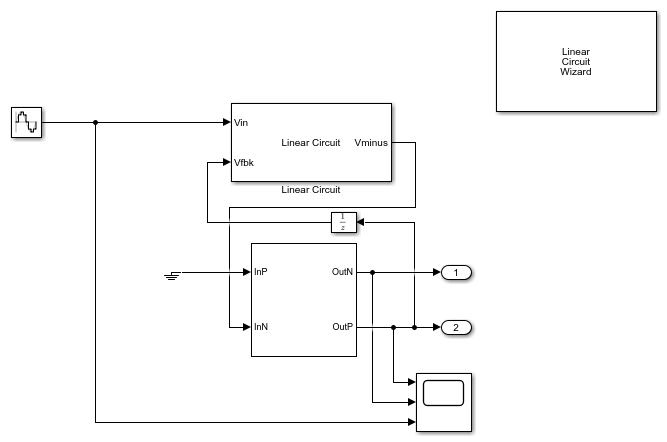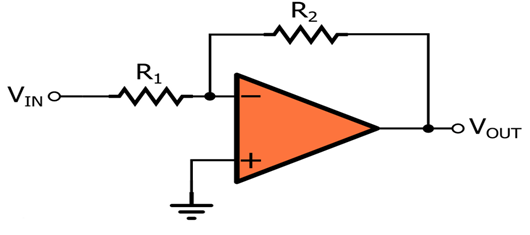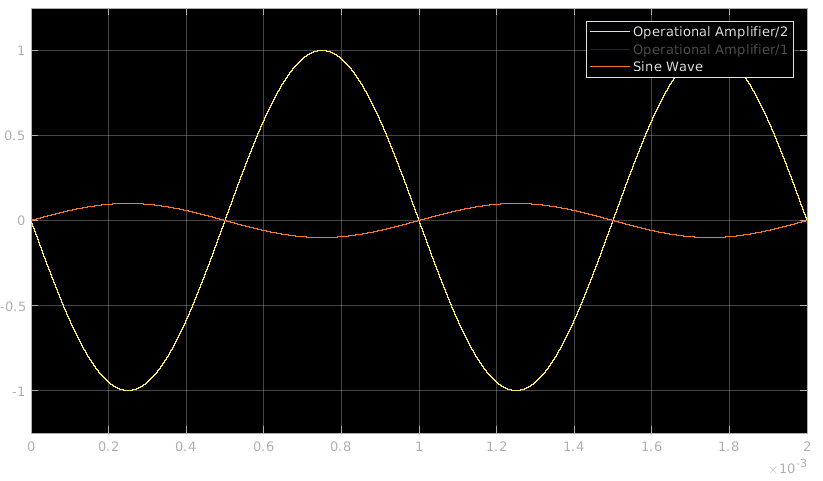This example shows how to model an inverting amplifier circuit using the Operational Amplifier (Op-amp) block and Linear Circuit wizard (LCW).

Inverting Amplifier

An inverting amplifier circuit contains an Op-amp and a feedback network consisting of passive resistance values. The inverting amplifier in this example is modeled using the Operational Amplifier block and the Linear Circuit Wizard to model the feedback network. The Linear Circuit Wizard uses a SPICE netlist describing the feedback stage from the output of the Op-amp to its input.

Open the model Inverting_Amplifier attached to this example.

open_system('Inverting_Amplifier');Operational Amplifier

The Operationa Amplifier block is based on a System object™ that takes in input parameters from a mask. The Op-amp can be modeled in two ways:

1) Double Pole Circuit: Circuit parameters are entered that translate to a double pole circuit.2) Multiple Pole Circuit: Transfer function poles and zeros are entered to model any Op-amp circuit.Linear Circuit Wizard

The Linear Circuit Wizard block can take in a SPICE netlist and generate a linear circuit block. You can specify the SPICE netlist file name and click on the Build/modify block button to create a circuit block that models the SPICE netlist.Operation

The inverting amplifier circuit is designed with an Op-amp and a feedback network consisting of two resistors R2 and R1.The feedback from Op-amp output to the input terminal is modeled as a SPICE file (R2 = 20KOhms, R1 = 2KOhms).The closed loop gain of the inverting amplifier is -R2/R1, which is equal to -10. The input stimuli given to this model is sampled signal and hence a sample rate has to be specified. The same sample scheme can be maintained at the Op-amp block as well.An input signal of 0.1V sine wave with 1KHz frequency is provided to the circuit model. The input and corresponding output are seen on the scope.